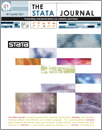Home  >>  Archives  >>  Volume 13 Number 3  >>  st0308

#### The Stata JournalVolume 13 Number 3: pp. 547-573

Subscribe to the Stata Journal## Copula-based maximum-likelihood estimation of sample-selection models

 Takuya Hasebe Graduate Center, City University of New York New York, NY thasebe@gc.cuny.edu
Abstract.  Sample-selection issues are common problems in empirical studies of labor economics and other applied microeconomics. A common estimation method is maximum likelihood estimation under the assumption of joint normality. It is well known, however, that the violation of distributional assumptions leads to inconsistency of a maximum likelihood estimator. Early work on sample-selection models that relaxes the normality assumption was done by Lee (1983, 1984). His approach was to transform nonnormal disturbances in the models into normal variates that are then assumed to be jointly normally distributed. As we will see, this is a special case of the copula approach that Smith (2003) applies to sample-selection models. The copula approach adds more flexibility to model specifications.

In this article, I discuss the maximum likelihood estimation of sample-selection models with the copula approach to relax the assumption of joint normality. Although there are several types of sample-selection models, I discuss two in particular: a bivariate sample-selection model and an endogenous switching regression model. I also introduce the Stata commands heckmancopula and switchcopula, which implement the estimation of each model, respectively.

View all articles by this author: Takuya Hasebe

View all articles with these keywords: heckmancopula, switchcopula, copula method, sample-selection models